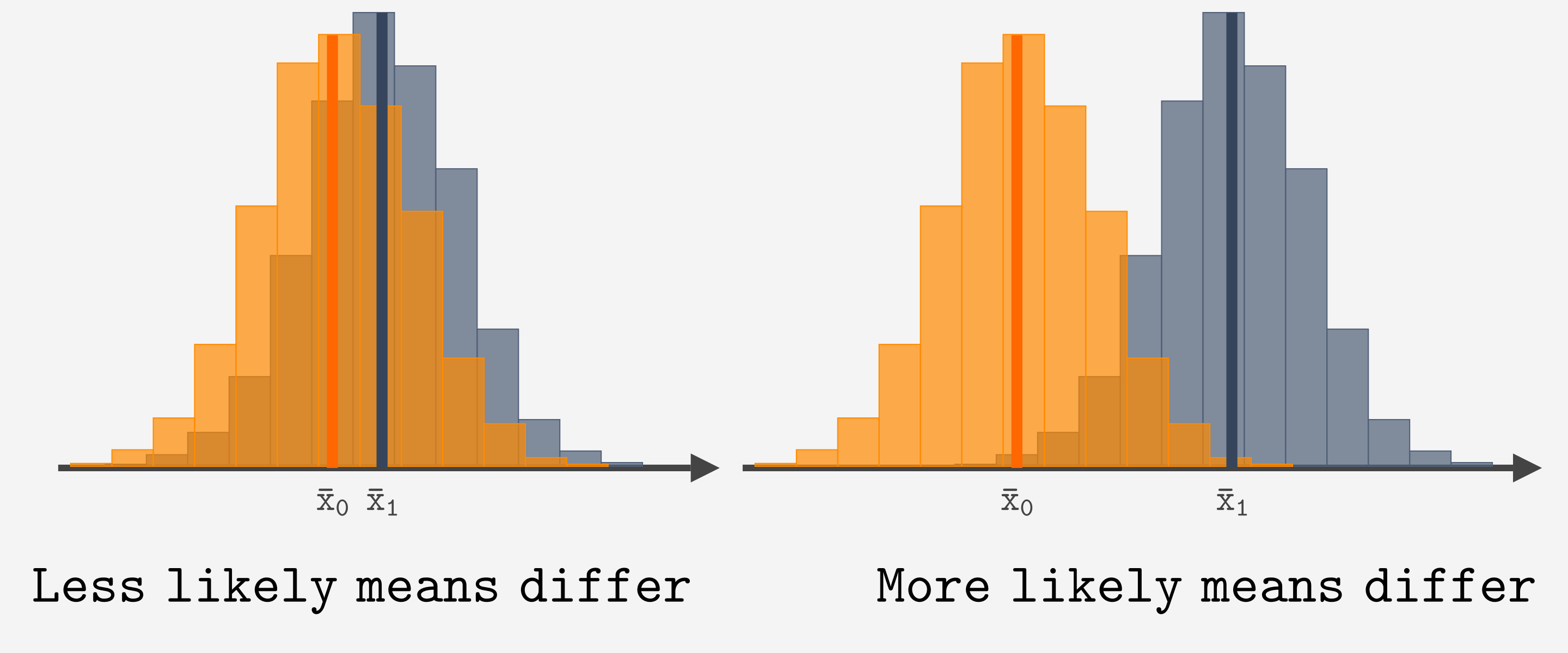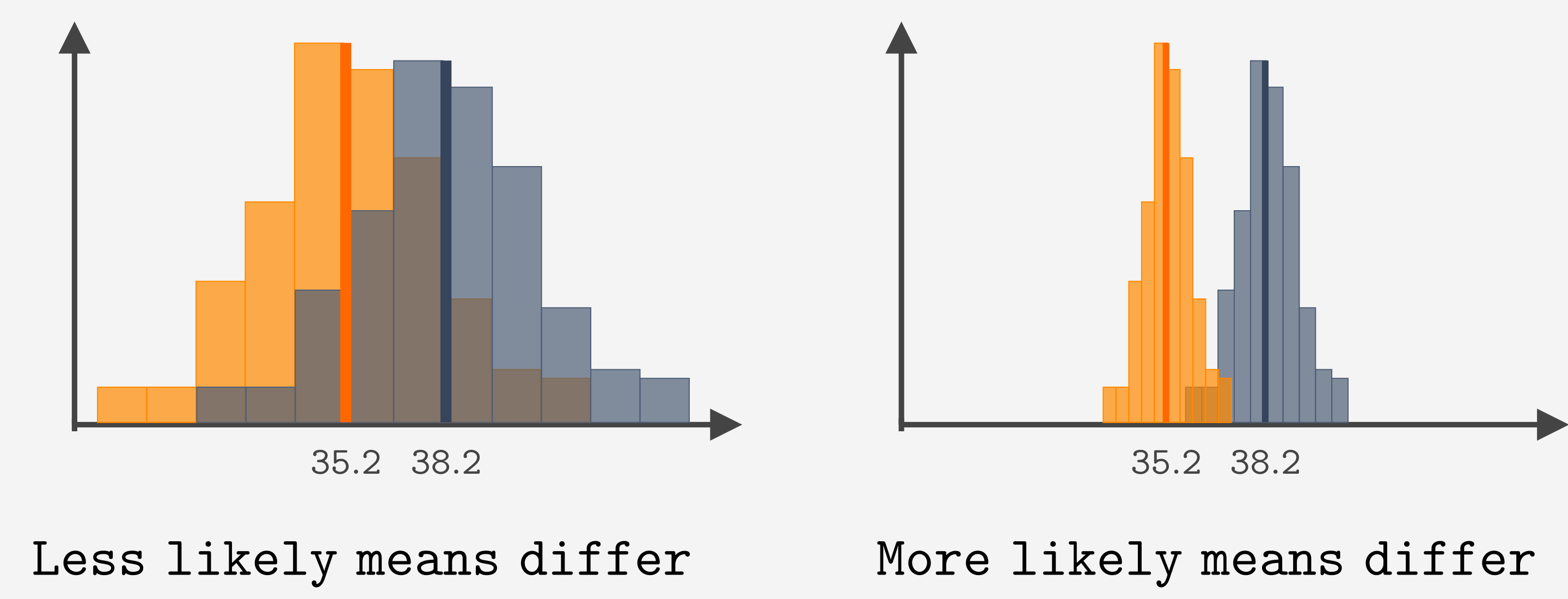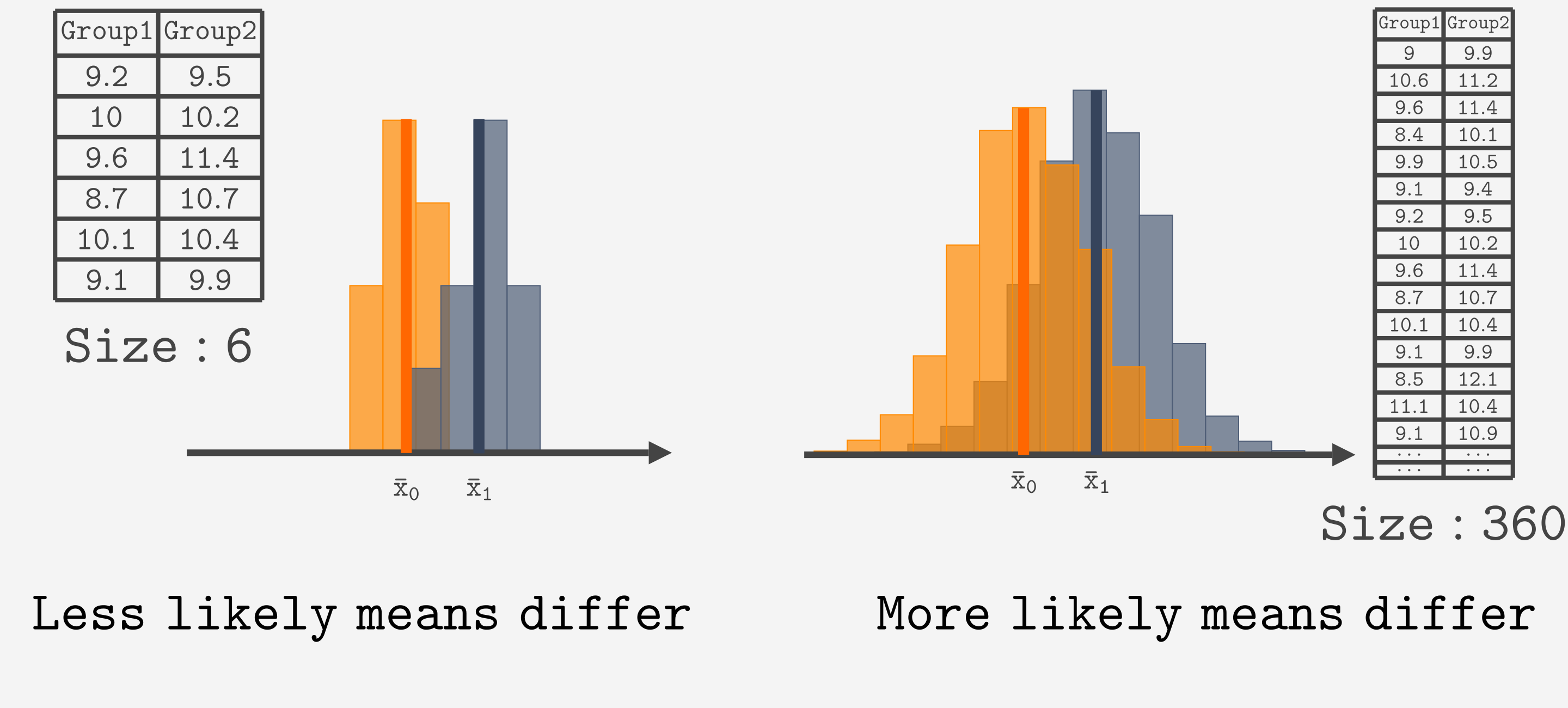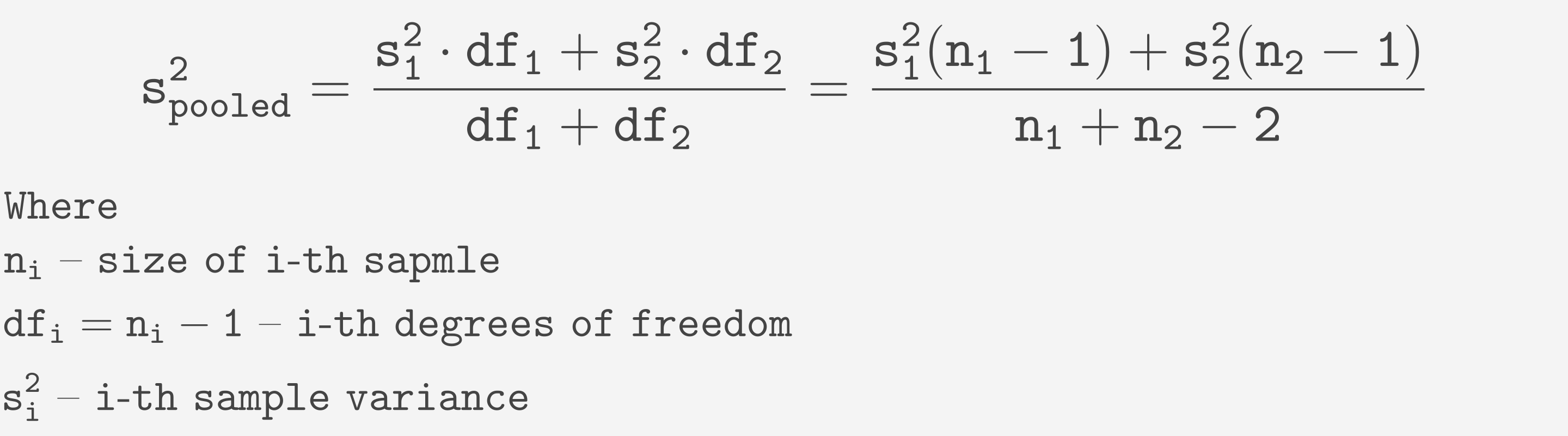Course Content

# Learning Statistics with Python

Learning Statistics with Python

##t-test Mathematically

The task of the t-test is to determine whether the difference between the two samples' means is significant. What should we take into consideration to perform it?

• Obviously, we should consider the difference between the means itself.• As shown in the image below, the variance matters too!• Also, the size of each sample should be taken into consideration.To account for the difference between the means, we simply calculate that difference:The situation becomes more complex when it comes to variance. The t-test assumes that the variance is equal for both samples. We will delve deeper into this in the t-test assumptions chapter. To estimate the variance from two samples, the pooled variance formula is applied:And to account for the size, we need sample sizes:Let's put it all together into t statistic.You may have noticed that sample sizes are not used in the most intuitive manner. However, this approach ensures that t follows the t-distribution, as we'll explore in the next chapter.

#What sample properties does the t-test take into account?

## Select a few correct answers

Everything was clear?

Section 6. Chapter 3